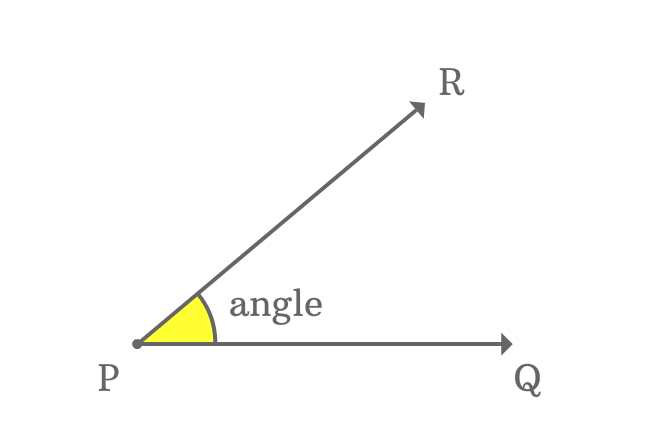# Sides $($or$)$ Arms of an angle

The lines which form angle an angle by their intersection at a point are called the sides of an angle. They are also called as arms of an angle.

## Example$\overrightarrow{PQ}$ and $\overrightarrow{PR}$ are two lines and they both have a common $P$ where an angle is made.

The lines $\overrightarrow{PQ}$ and $\overrightarrow{PR}$ are reason for the forming an angle. So, the lines $\overrightarrow{PQ}$ and $\overrightarrow{PR}$ are called the sides of an angle and they are called a arms of an angle.

Latest Math Topics
Jun 26, 2023
Jun 23, 2023

###### Math Questions

The math problems with solutions to learn how to solve a problem.

Learn solutions

Practice now

###### Math Videos

The math videos tutorials with visual graphics to learn every concept.

Watch now

###### Subscribe us

Get the latest math updates from the Math Doubts by subscribing us.